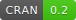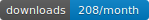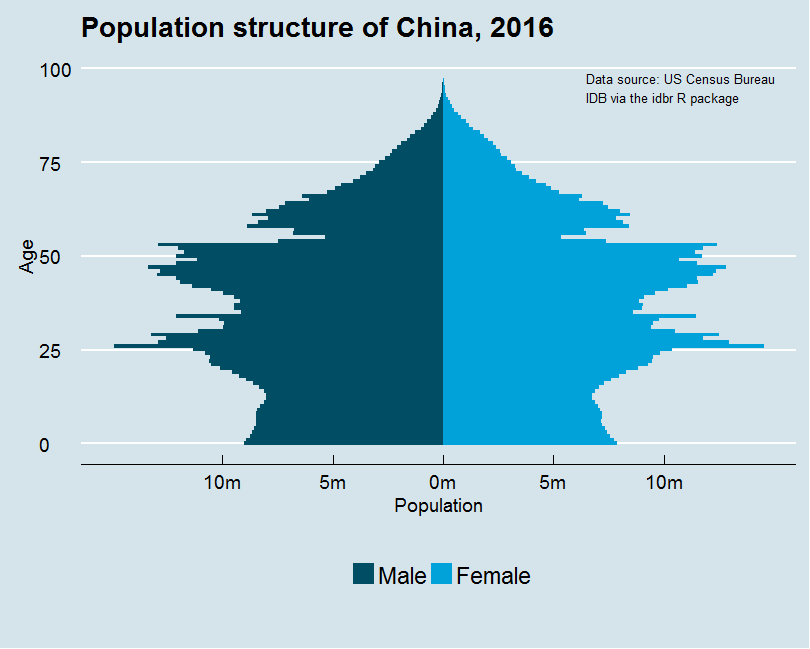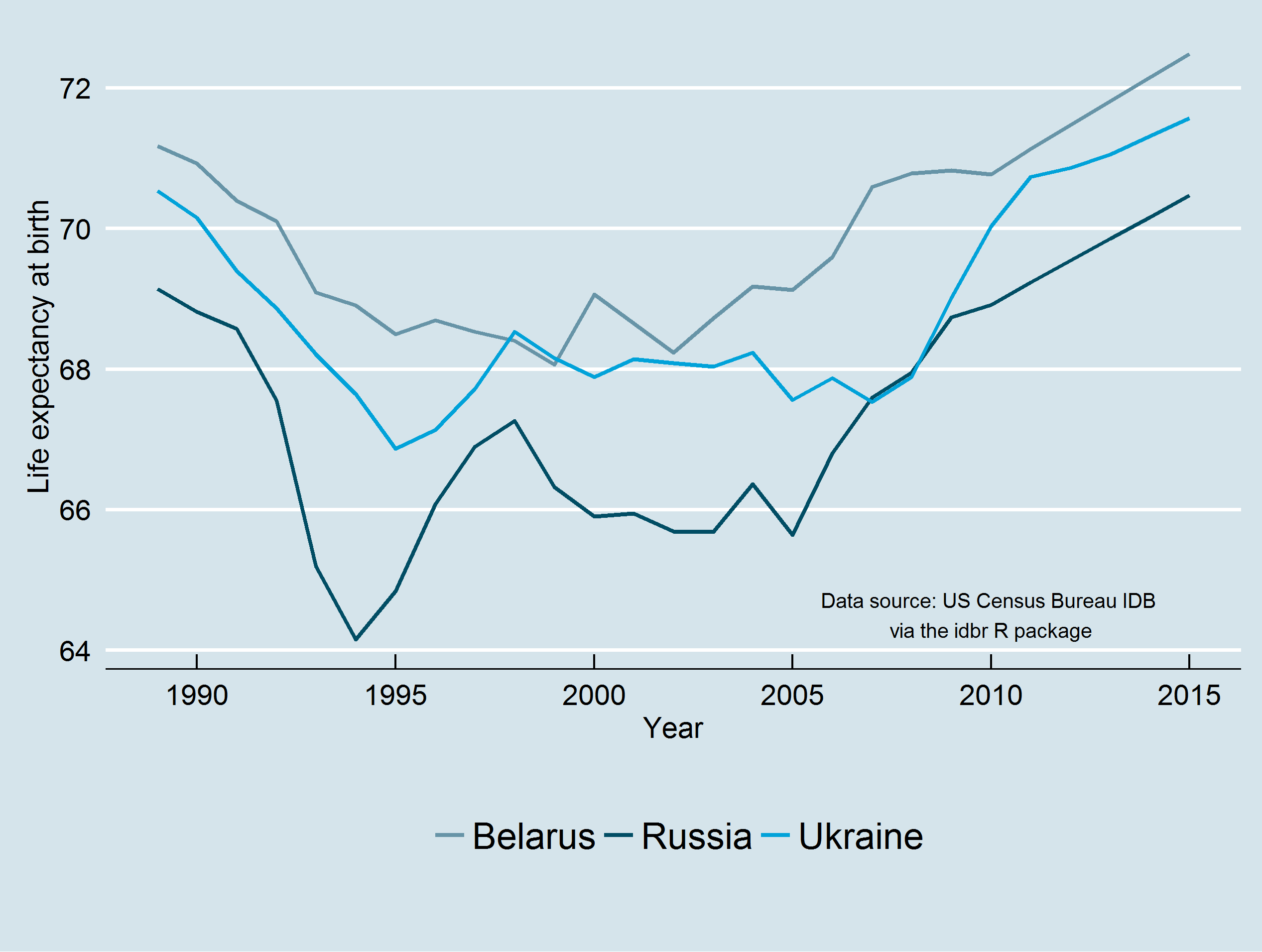# idbr

An R interface to the US Census Bureau International Data Base APIThis R package enables users to fetch international demographic indicators from the US Census Bureau’s International Data Base API and return R data frames. Total population data are available from 1950-2050 (projected); age-group subsets and other demographic indicators are available for varying periods by country.

Install from CRAN with:

``install.packages('idbr')``

Or get the development version from GitHub:

``````library(devtools)
install_github('walkerke/idbr')``````

## Basic usage:

To get started, load idbr and set your Census API key with the `idb_api_key()` function. An API key can be obtained from the US Census Bureau at http://api.census.gov/data/key_signup.html.

``````library(idbr)

idb_api_key('Your API key goes here')``````

There are two main functions in the idbr package: `idb1()` and `idb5()`. `idb1()` grants access to the single-year-of-age dataset, which returns population counts by single-year age bands, subsetted optionally by sex and age range. This dataset is well-suited for analyses and visualizations of the age composition of countries, such as population pyramids:

``````library(dplyr)
library(ggplot2)
library(ggthemes)

# Supply the FIPS country code and year for which you'd like to request data, optionally by sex
male <- idb1('China', 2016, sex = 'male') %>%
mutate(POP = POP * -1,
SEX = 'Male')

female <- idb1('China', 2016, sex = 'female') %>%
mutate(SEX = 'Female')

china <- rbind(male, female)

# Build the visualization

ggplot(china, aes(x = AGE, y = POP, fill = SEX, width = 1)) +
coord_flip() +
annotate('text', x = 95, y = 6500000,
label = 'Data source: US Census Bureau \nIDB via the idbr R package',
size = 3.5, hjust = 0) +
geom_bar(data = subset(china, SEX == "Female"), stat = "identity") +
geom_bar(data = subset(china, SEX == "Male"), stat = "identity") +
scale_y_continuous(breaks = seq(-10000000, 10000000, 5000000),
labels = paste0(as.character(c(seq(10, 0, -5), c(5, 10))), "m")) +
theme_economist(base_size = 14) +
scale_fill_economist() +
ggtitle('Population structure of China, 2016') +
ylab('Population') +
xlab('Age') +
theme(legend.position = "bottom",
legend.title = element_blank()) +
guides(fill = guide_legend(reverse = TRUE))``````The `idb5()` function grants access to the five-year-age-band dataset, which includes a wide range of fertility, mortality, and migration indicators along with overall population counts. In turn, it can be used to inform analyses of how demographic indicators vary by country over time, among many other use cases.

``````# Fetch data for 'E0', which represents life expectancy at birth
ssr_df <- idb5(c('Russia', 'Ukraine', 'Belarus'), 1989:2015,
variables = 'E0', country_name = TRUE)

ggplot(ssr_df, aes(x = time, y = E0, color = NAME)) +
geom_line(size = 1) +
theme_economist(base_size = 14) +
scale_color_economist() +
ylab('Life expectancy at birth') +
xlab('Year') +
theme(legend.title = element_blank(),
legend.position = "bottom") +
annotate('text', x = 2010, y = 64.5,
label = 'Data source: US Census Bureau IDB \nvia the idbr R package',
size = 3.5)``````